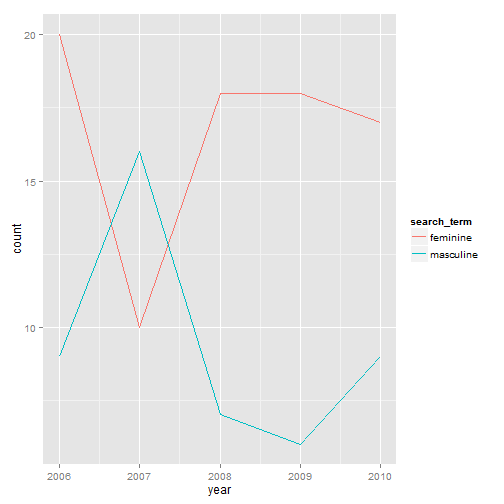This is a tutorial on how to download data from NY Times.

The New York Times is a widely circulated newspaper relied upon by many for reputable news. Recently, the Times has developed an Application Programming Interface (API) to allow people to download bibliometric data.

One aspect of the bibliometric data available for download are counts of words. The Times has indexed words that appear in its database of articles, including news items, book reviews, and opinions. Full documentation of the API is here:

http://developer.nytimes.com/

It is possible to search for, download, and analyze these data for use in research.

This tutorial will demonstrate how to accomplish this with R. In particular, we will search for the terms 'masculine' and 'feminine' in the opinion articles from 2006 to 2010. We will then graph how many times these two terms have been mentioned in the opinion sections over time.

Create a dataframe to house the data.

We will first create a dataframe to house the data. We will start off with a dataframe that has one column, and 5 rows - equal to the number of years from 2006 to 2010.

``````data <- as.data.frame(matrix(ncol=1, nrow=5))
``````

Create a column of years.

Next, we will list out the years in sequence, and rename the column to “year”

``````data\$V1 <- seq(from=2006, to=2010)

names(data)[names(data)=="V1"] <- "term"
``````

Specify the terms to search for in the NYT.

``````search_terms <- c("masculine", "feminine")
``````

Loop through those search terms and pull in NYT data.

``````# The NY Times API returns results in JSON. We will load some necessary packages to parse that data.

library(jsonlite)

# Create a loop
for(i in 1:length(search_terms)){

# Get each search term and store those in objects
term <- search_terms[i]

# Download and parse the data, using a URL to query NY Times.
# I have removed my key in the code below, please substitute your own.

x <- fromJSON(paste("http://api.nytimes.com/svc/search/v2/articlesearch.json?q=%22'", term,
"%22&fq=section_name:%22Opinion%22%20AND%20source:(%22The%20New%20York%20Times%22)&
begin_date=20060101&end_date=20101231&facet_field=pub_year&facet_filter=true&
callback=svc_search_v2_articlesearch&api-key=REDACTED", sep=""))

# Create data from resulting output
temp <- as.data.frame(x\$response\$facets\$pub_year\$terms)

# Merge NYT data with dataframe created step 1, matching by years
data <- merge(data, temp, by ="term", all.x=TRUE)

# Remove temporary dataframe
rm(temp)

# Reaname column by search term
colname <- paste(term, sep="")

# Rename added column with ID
names(data)[names(data)=="count"] <- colname
}

# Rename the year variable from "term" to "year"
names(data)[names(data)=="term"] <- "year"
``````

A look at the data.

``````head(data)
``````
``````##   year masculine feminine
## 1 2006         9       20
## 2 2007        16       10
## 3 2008         7       18
## 4 2009         6       18
## 5 2010         9       17
``````

Reshape the data to put it in a graph friendly (long) format.

``````data_long <- reshape(data,
varying = c("feminine", "masculine"),
v.names = "count",
timevar = "search_term",
times = c("feminine", "masculine"),
direction = "long")
``````

Graph the data to observe trends over time.

As you can see from the graph below, opinion articles have mentioned “feminine” more frequently than “masculine” throughout years, with an exception during 2007.``````library(ggplot2)

p <- ggplot(data_long, aes(x=year, y=count, group=search_term))

p +  geom_line(aes(colour = search_term))
``````